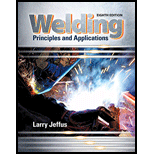Chapter 22, Problem 2R### Welding: Principles and Applicatio...

8th Edition
Larry Jeffus
ISBN: 9781305494695

#### Solutions

Chapter
Section### Welding: Principles and Applicatio...

8th Edition
Larry Jeffus
ISBN: 9781305494695
Textbook Problem
631 views

# What stresses must a welded joint withstand?

To determine

The types of stresses a welded joint must withstand.

Explanation

A welded joint withstands following stresses:

1. Tensile stress
2. Compressive stress
3. Bending stress
4. Torsion stress, and
5. Shear stress
1. Tensile stress is defined as a quantity related to tensile force acting upon an area. There is application of stretching force.
2.

3. Compressive stress is defined as the opposite of tensile stress. A stress generated on applying squeezing force on an object is called compressive stress.
4.

5. Bending stresses are defined as the stress generated by the application of a point load...

### Still sussing out bartleby?

Check out a sample textbook solution.

See a sample solution

#### The Solution to Your Study Problems

Bartleby provides explanations to thousands of textbook problems written by our experts, many with advanced degrees!

Get Started